﻿ 三支决策的时空性
«上一篇文章快速检索 高级检索

 智能系统学报2019, Vol. 14Issue (1): 141-149  DOI: 10.11992/tis.2018040450

### 引用本文LIU Dun, LI Tianrui, LIANG Decui, et al. Temporality and spatiality of three-way decisions[J]. CAAI Transactions on Intelligent Systems, 2019, 14(1): 141-149. DOI: 10.11992/tis.201804045.### 文章历史

1. 西南交通大学 经济管理学院，四川 成都 610031;
2. 西南交通大学 信息科学与技术学院，四川 成都 610031;
3. 电子科技大学 经济与管理学院，四川 成都 610054

Temporality and spatiality of three-way decisions
LIU Dun 1, LI Tianrui 2, LIANG Decui 3, YANG Xin 2
1. School of Economics and Management, Southwest Jiaotong University, Chengdu 610031, China;
2. School of Information Science and Technology, Southwest Jiaotong University, Chengdu 610031, China;
3. School of Management and Economics, University of Electronic Science and Technology of China, Chengdu 610054, China
Abstract: In this paper, the development history and recent researches of three-way decisions (3WDs) are discussed, and two models, each of temporal 3WDs and spatial 3WDs, are proposed based on the summary and analysis of theories, methods, algorithms, and applications of 3WD. The temporal 3WDs focus on the sequential decisions under dynamic decision environment, and the spatial 3WDs emphasize the optimal granular selection with " multi-level” and " multi-view” strategies. In addition, the temporality and spatiality of 3WDs are investigated and analyzed in depth, clarifying the development process and research context of 3WDs. Finally, this paper presents the research status and future research directions of 3WD.
Key words: three-way decisions    granular computing    temporal three-way decisions    spatial three-way decisions    rough set theory    granular analysis    multi-level    multi-view

1 三支决策模型与基本概念

 $f:U \to \left\{ {{R_1},\;{R_2},\;{R_3}} \right\}$ (1)

 $\begin{array}{l} R_1^c = {R_2} \cup {R_3}\\ R_2^c = {R_1} \cup {R_3}\\ R_3^c = {R_1} \cup {R_2} \end{array}$ (2)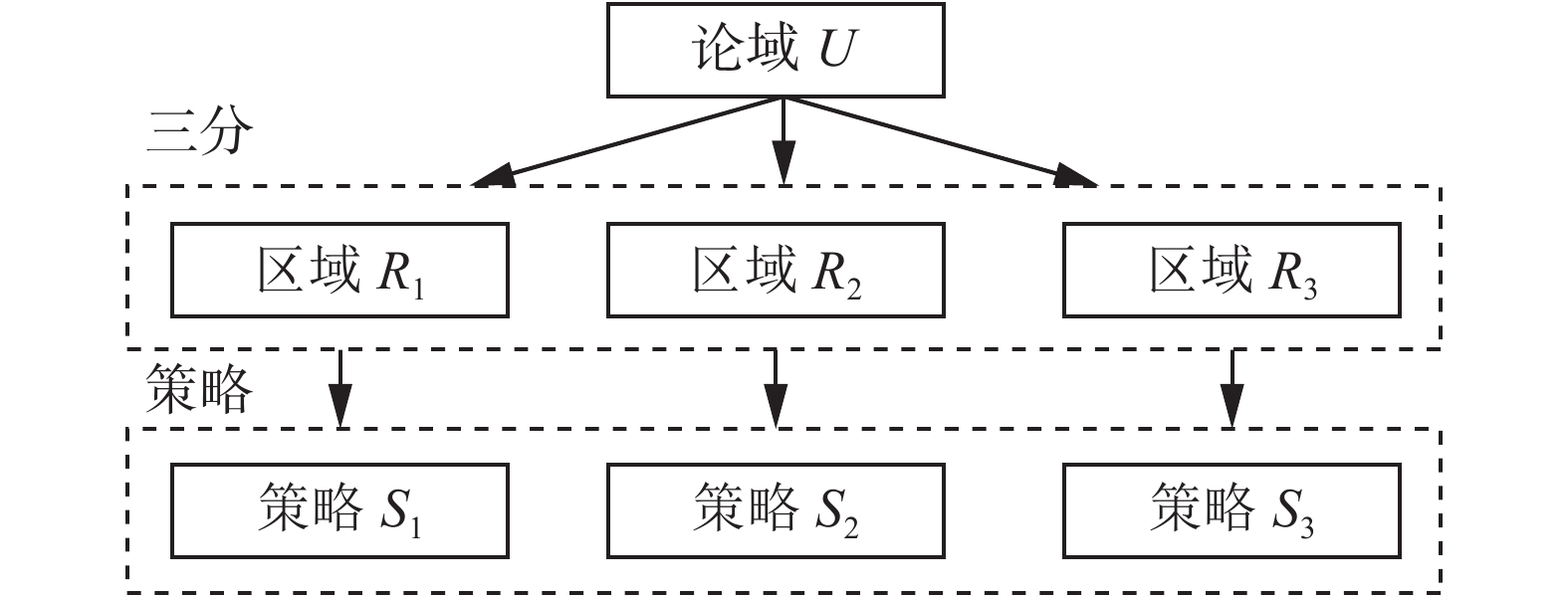Download: 图 1 三支决策模型示意图 Fig. 1 Three-way decision model diagram表 1 不同情形下的三支决策模型 Tab.1 The three-way decision models under different conditions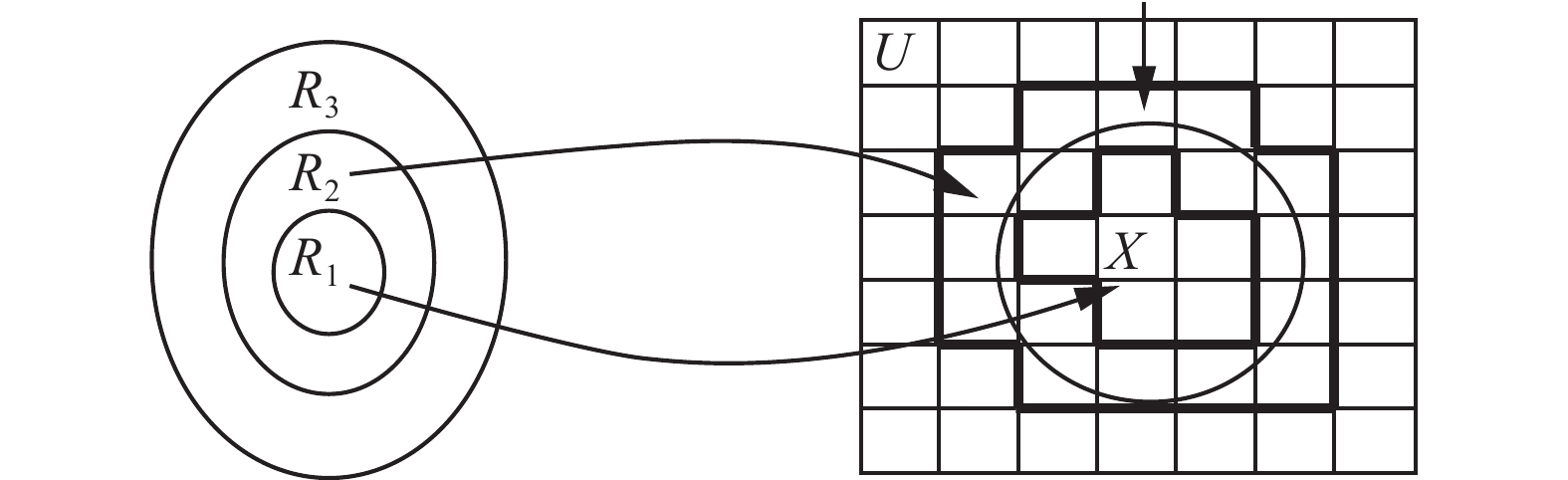Download: 图 2 三支决策与粗糙集理论的转换模型 Fig. 2 Transformation model between 3WD and rough sets

2 基于时间维度三支决策模型

2001年7月13日，国际奥委会第112次全会在俄罗斯莫斯科召开，与会全体委员将投票选举出第29届夏季奥运会的举办城市。有北京、多伦多、伊斯坦布尔、巴黎和大阪5个候选城市参加选举，一共118名国际奥委会委员进行投票。在第一轮投票中，有14名申办城市委员和时任主席萨马兰奇不参加投票，实际有104名委员投票，2票弃权，有效票102张。其中，北京获得44票，多伦多获得20票，伊斯坦布尔获得17票，巴黎获得15票，大阪获得6票。第一轮投票结束，北京占据优势，大阪得票最少被淘汰，其余3个城市作为待定城市进入下一轮竞选。在接下来的第二轮投票中，有106名委员(2名日本委员因大阪淘汰获得投票权)实际参加投票，1票弃权，有效票105张。最终，北京获得56票，超半数票成功获得举办权，多伦多、巴黎、伊斯坦布尔分别获得22票、18票和9票被淘汰。从投票过程可以看到，随着外界环境和时间的变化，中选城市( ${R_1}$ 域)、候选城市( ${R_2}$ 域)和落选城市( ${R_3}$ 域)也会随之发生改变。表 2 Savage可能采取的3种策略和后果 Tab.2 Three strategies and results of Savage’s omelet problem

 ${R^{\left( t \right)}}\left( {{s_j}|x} \right) = \sum\limits_{i = 1}^m {{\lambda ^{\left( t \right)}}\left( {{s_j}|{\omega _i}} \right){P^{\left( t \right)}}\left( {{\omega _i}|x} \right)}$ (3)

 $\left\{ {\begin{array}{*{20}{l}} {{R^{\left( t \right)}}\left( {{s_1}|x} \right){\rm{ = }}{\lambda ^{\left( t \right)}}\left( {{s_1}|{\omega _1}} \right){P^{\left( t \right)}}\left( {{\omega _1}|x} \right) + {\lambda ^{\left( t \right)}}\left( {{s_1}|{\omega _2}} \right){P^{\left( t \right)}}\left( {{\omega _2}|x} \right)}\\ {{R^{\left( t \right)}}\left( {{s_2}|x} \right){\rm{ = }}{\lambda ^{\left( t \right)}}\left( {{s_2}|{\omega _1}} \right){P^{\left( t \right)}}\left( {{\omega _1}|x} \right) + {\lambda ^{\left( t \right)}}\left( {{s_2}|{\omega _2}} \right){P^{\left( t \right)}}\left( {{\omega _2}|x} \right)}\\ {{R^{\left( t \right)}}\left( {{s_3}|x} \right){\rm{ = }}{\lambda ^{\left( t \right)}}\left( {{s_3}|{\omega _1}} \right){P^{\left( t \right)}}\left( {{\omega _1}|x} \right) + {\lambda ^{\left( t \right)}}\left( {{s_3}|{\omega _2}} \right){P^{\left( t \right)}}\left( {{\omega _2}|x} \right)} \end{array}} \right.$ (4)

1)若 ${R^{\left( t \right)}}\left( {{s_1}|x} \right) \leqslant {R^{\left( t \right)}}\left( {{s_2}|x} \right)$ ${R^{\left( t \right)}}\left( {{s_1}|x} \right) \leqslant {R^{\left( t \right)}}\left( {{s_3}|x} \right)$ 同时成立，则对 $x$ 采取 ${S_1}$ 策略；

2)若 ${R^{\left( t \right)}}\left( {{s_2}|x} \right) \leqslant {R^{\left( t \right)}}\left( {{s_1}|x} \right)$ ${R^{\left( t \right)}}\left( {{s_2}|x} \right) \leqslant {R^{\left( t \right)}}\left( {{s_3}|x} \right)$ 同时成立，则对 $x$ 采取 ${S_{\rm{2}}}$ 策略；

3)若 ${R^{\left( t \right)}}\left( {{s_3}|x} \right) \leqslant {R^{\left( t \right)}}\left( {{s_1}|x} \right)$ ${R^{\left( t \right)}}\left( {{s_3}|x} \right) \leqslant {R^{\left( t \right)}}\left( {{s_2}|x} \right)$ 同时成立，则对 $x$ 采取 ${S_{\rm{3}}}$ 策略。

 $\left\{ \begin{array}{l} {\lambda ^{(t)}}({s_{\rm{1}}}|{\omega _{\rm{1}}}) \leqslant {\lambda ^{(t)}}({s_{\rm{2}}}|{\omega _{\rm{1}}}) < {\lambda ^{(t)}}({s_{\rm{3}}}|{\omega _{\rm{1}}})\\ {\lambda ^{(t)}}({s_{\rm{3}}}|{\omega _{\rm{2}}}) \leqslant {\lambda ^{(t)}}({s_{\rm{2}}}|{\omega _{\rm{2}}}) < {\lambda ^{(t)}}({s_{\rm{1}}}|{\omega _{\rm{2}}}) \end{array}\right.$ (4)

1) 若 ${P^{(t)}}({\omega _{\rm{1}}}|x) \geqslant {\alpha ^{(t)}}$ ，则 $x$ ${\omega _{\rm{1}}}$ 下采取策略 ${S_1}$

2) 若 ${\beta ^{(t)}} \leqslant {P^{(t)}}({\omega _{\rm{1}}}|x) \leqslant {\alpha ^{(t)}}$ ，则 $x$ ${\omega _{\rm{1}}}$ 下采取策略 ${S_{\rm{2}}}$

3) 若 ${P^{(t)}}({\omega _{\rm{1}}}|x) \leqslant {\beta ^{(t)}}$ ，则 $x$ ${\omega _{\rm{1}}}$ 下采取策略 ${S_{\rm{3}}}$

 $\left\{ \begin{array}{l} {\alpha ^{\left( t \right)}}{\rm{ = }}\displaystyle\frac{{ {{\lambda ^{\left( t \right)}}\left( {{s_1}|{\omega _2}} \right) - {\lambda ^{\left( t \right)}}\left( {{s_2}|{\omega _2}} \right)} }}{{\left[ {{\lambda ^{\left( t \right)}}\left( {{s_1}|{\omega _2}} \right) - {\lambda ^{\left( t \right)}}\left( {{s_2}|{\omega _2}} \right)} \right] + \left[ {{\lambda ^{\left( t \right)}}\left( {{s_2}|{\omega _1}} \right) - {\lambda ^{\left( t \right)}}\left( {{s_1}|{\omega _1}} \right)} \right]}}\\ {\beta ^{\left( t \right)}}{\rm{ = }}\displaystyle\frac{{ {{\lambda ^{\left( t \right)}}\left( {{s_2}|{\omega _2}} \right) - {\lambda ^{\left( t \right)}}\left( {{s_2}|{\omega _2}} \right)} }}{{\left[ {{\lambda ^{\left( t \right)}}\left( {{s_2}|{\omega _2}} \right) - {\lambda ^{\left( t \right)}}\left( {{s_3}|{\omega _2}} \right)} \right] + \left[ {{\lambda ^{\left( t \right)}}\left( {{s_3}|{\omega _1}} \right) - {\lambda ^{\left( t \right)}}\left( {{s_1}|{\omega _1}} \right)} \right]}}\\ {\gamma ^{\left( t \right)}}{\rm{ = }}\displaystyle\frac{{ {{\lambda ^{\left( t \right)}}\left( {{s_1}|{\omega _2}} \right) - {\lambda ^{\left( t \right)}}\left( {{s_3}|{\omega _2}} \right)} }}{{\left[ {{\lambda ^{\left( t \right)}}\left( {{s_1}|{\omega _2}} \right) - {\lambda ^{\left( t \right)}}\left( {{s_3}|{\omega _2}} \right)} \right] + \left[ {{\lambda ^{\left( t \right)}}\left( {{s_3}|{\omega _1}} \right) - {\lambda ^{\left( t \right)}}\left( {{s_1}|{\omega _1}} \right)} \right]}} \end{array}\right.$ (6)

1) 若 ${P^{(t)}}({\omega _{\rm{1}}}|x) \geqslant {\gamma ^{(t)}}$ ，则 $x$ ${\omega _{\rm{1}}}$ 下采取策略 ${S_1}$

2) 若 ${P^{(t)}}({\omega _{\rm{1}}}|x) < {\gamma ^{(t)}}$ ，则 $x$ ${\omega _{\rm{1}}}$ 下采取策略 ${S_{\rm{3}}}$

3 基于空间维度三支决策模型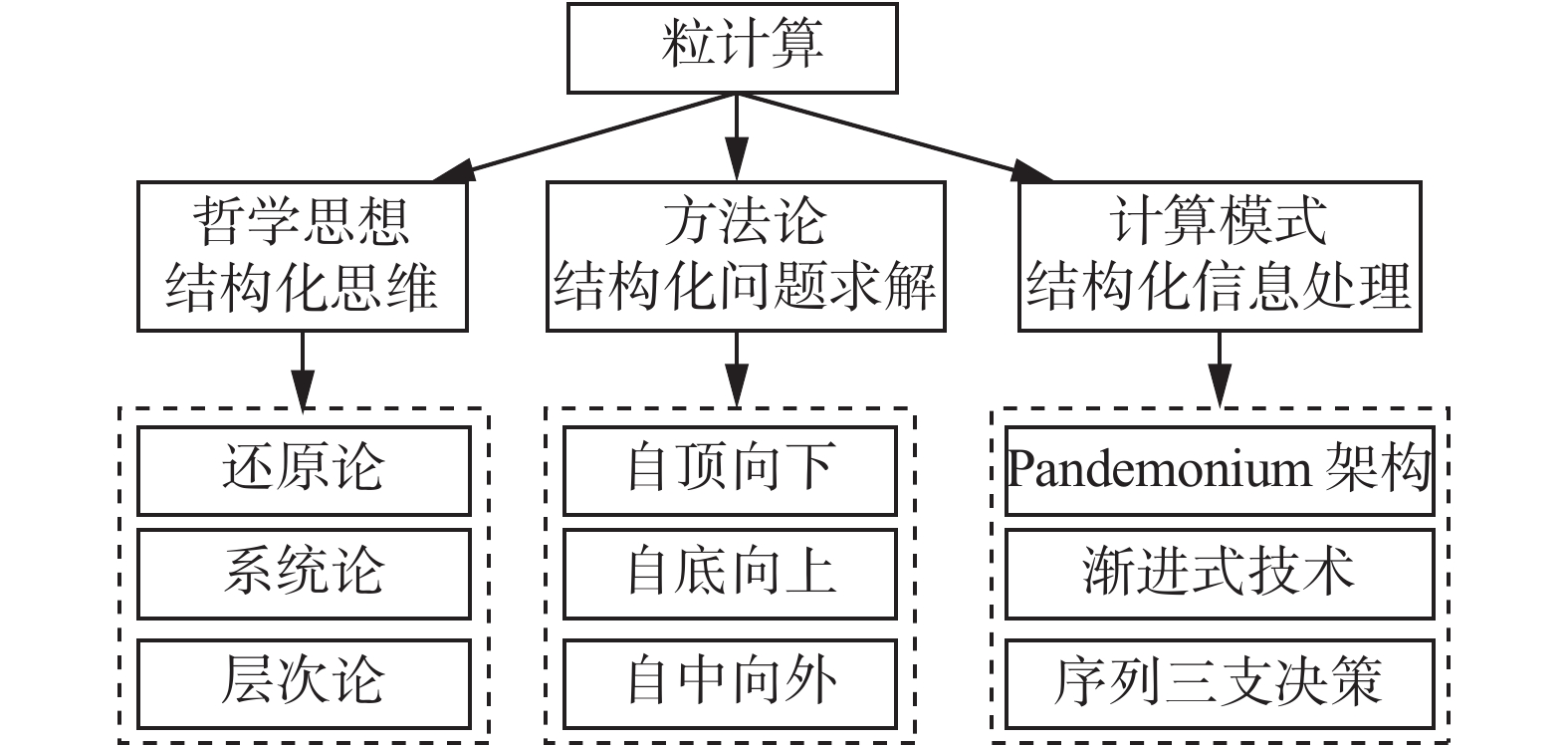Download: 图 3 粒计算的三元论 Fig. 3 Trialistic theory of granular computing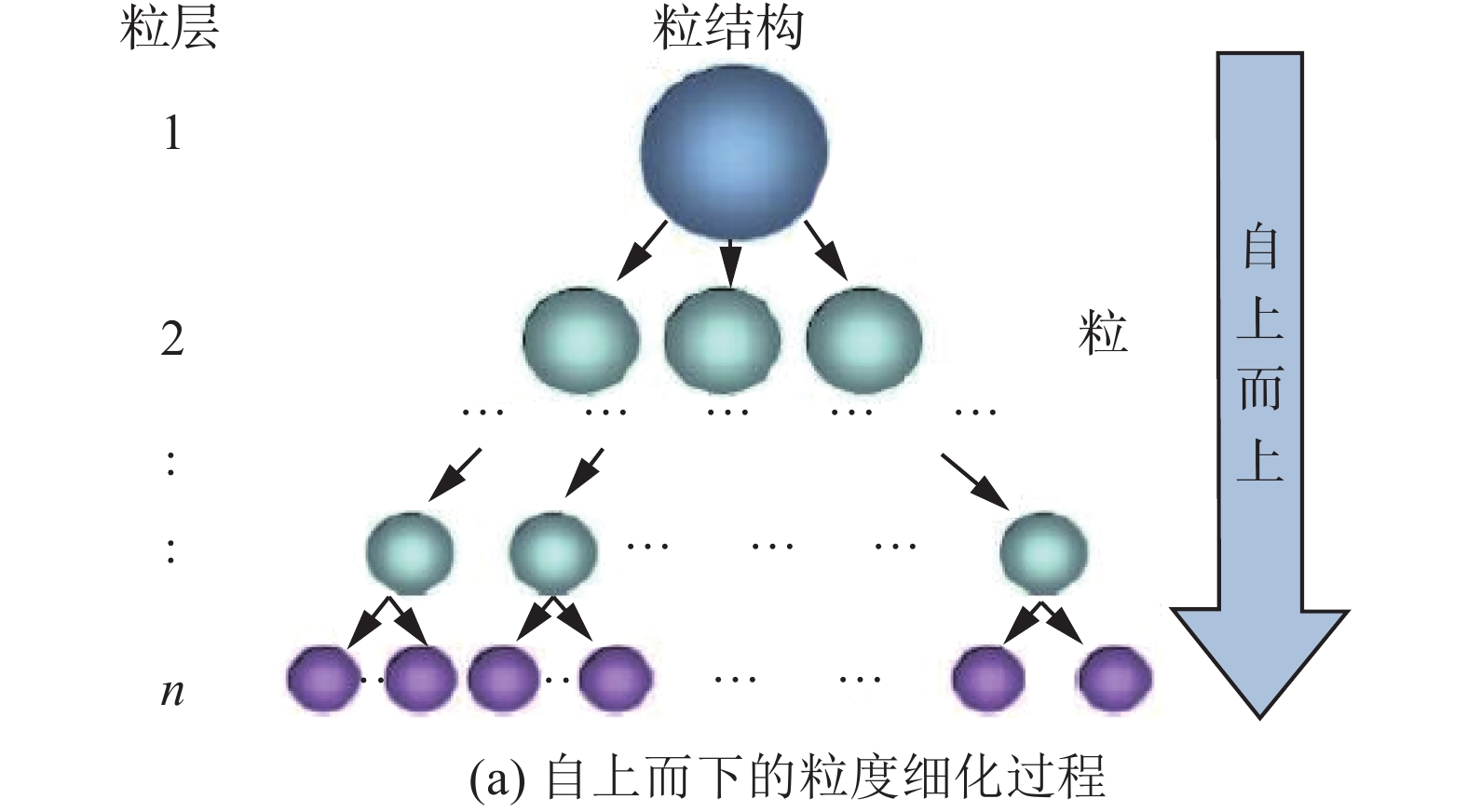Download: 图 4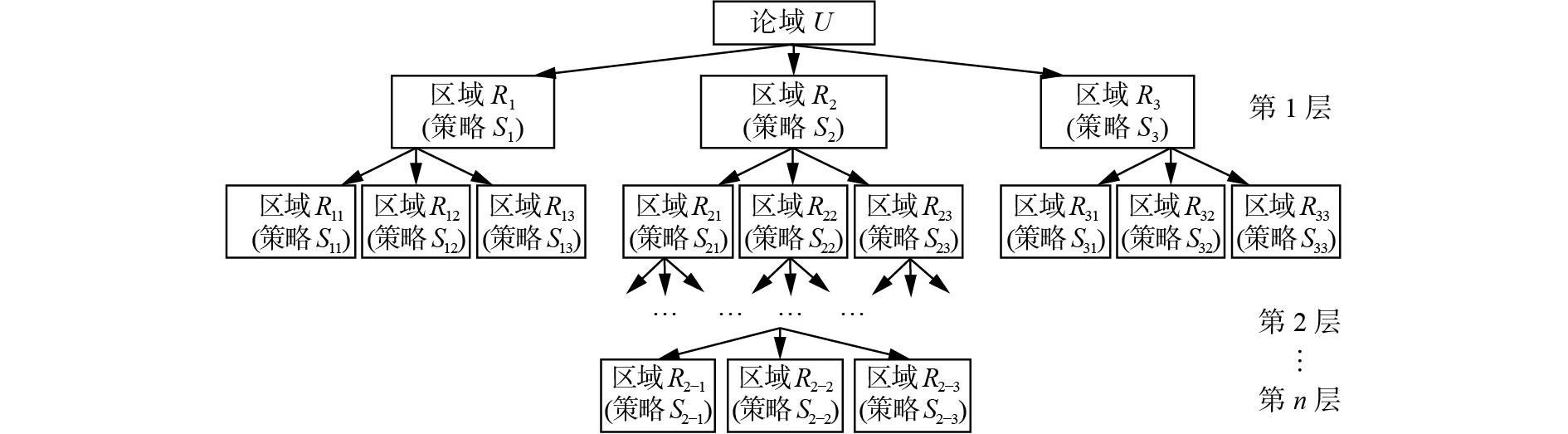Download: 图 5 空间三支决策一般模型 Fig. 5 General model of spatial three-way decisions

4 三支决策的时空性研究方法

5 结束语

  YAO Yiyu. Three-way decisions with probabilistic rough sets[J]. Information sciences, 2010, 180(3): 341-353. DOI:10.1016/j.ins.2009.09.021 (0)  HU Baoqing. Three-way decisions space and three-way decisions[J]. Information sciences, 2014, 281: 21-52. DOI:10.1016/j.ins.2014.05.015 (0)  LI Xiaonan, YI Huangjian, SHE Yanhong, et al. Generalized three-way decision models based on subset evaluation[J]. International journal of approximate reasoning, 2017, 83: 142-159. DOI:10.1016/j.ijar.2017.01.005 (0)  YAO Yiyu. Three-way decisions and cognitive computing[J]. Cognitive computation, 2016, 8(4): 543-554. DOI:10.1007/s12559-016-9397-5 (0)  CIUCCI D, DUBOIS D. A map of dependencies among three-valued logics[J]. Information science, 2013, 250: 162-177. DOI:10.1016/j.ins.2013.06.040 (0)  ABD EL-MONSEF M M E, KILANY N M. Decision analysis via granulation based on general binary relation[J]. International journal of mathematics and mathematical sciences, 2007: 12714. (0)  MA Weimin, SUN Bingzhen. On relationship between probabilistic rough set and Bayesian risk decision over two universes[J]. International journal of general systems, 2012, 41(3): 225-245. DOI:10.1080/03081079.2011.634067 (0)  QIAN Yuhua, ZHANG Hu, SANG Yanli, et al. Multigranulation decision-theoretic rough sets[J]. International journal of approximate reasoning, 2014, 55(1): 225-237. DOI:10.1016/j.ijar.2013.03.004 (0)  DENG Xiaofei, YAO Yiyu. Decision-theoretic three-way approximations of fuzzy sets[J]. Information sciences, 2014, 279: 702-715. DOI:10.1016/j.ins.2014.04.022 (0)  QI Jianjun, QIAN Ting, WEI Ling. The connections between three-way and classical concept lattices[J]. Knowledge-based systems, 2016, 91: 143-151. DOI:10.1016/j.knosys.2015.08.006 (0)  ZHANG Qinghua, XIA Deyou, WANG Guoyin. Three-way decision model with two types of classification errors[J]. Information sciences, 2017, 420: 431-453. DOI:10.1016/j.ins.2017.08.066 (0)  LIU Dun, LI Tianrui, RUAN Da. Probabilistic model criteria with decision-theoretic rough sets[J]. Information sciences, 2011, 181(17): 3709-3722. DOI:10.1016/j.ins.2011.04.039 (0)  LI Huaxiong, ZHOU Xianzhong. Risk decision making based on decision-theoretic rough set: a three-way view decision model[J]. International journal of computational intelligence systems, 2011, 4(1): 1-11. DOI:10.1080/18756891.2011.9727759 (0)  HERBERT J P, YAO Jingtao. Game-theoretic rough sets[J]. Fundamenta informaticae, 2011, 108(3/4): 267-286. (0)  YANG Xiaoping, YAO Jingtao. Modeling multi-agent three-way decisions with decision-theoretic rough sets[J]. Fundamenta informaticae, 2012, 115(2/3): 157-171. (0)  LIANG Decui, LIU Dun, PEDRYCZ W, et al. Triangular fuzzy decision-theoretic rough sets[J]. International journal of approximate reasoning, 2013, 54(8): 1087-1106. DOI:10.1016/j.ijar.2013.03.014 (0)  LIANG Decui, LIU Dun. Systematic studies on three-way decisions with interval-valued decision-theoretic rough sets[J]. Information sciences, 2014, 276: 186-203. DOI:10.1016/j.ins.2014.02.054 (0)  LIANG Decui, LIU Dun. Deriving three-way decisions from intuitionistic fuzzy decision-theoretic rough sets[J]. Information sciences, 2015, 300: 28-48. DOI:10.1016/j.ins.2014.12.036 (0)  YAO Yiyu, DENG Xiaofei. Sequential three-way decisions with probabilistic rough sets[C]//Proceeding of 10th International Conference on Cognitive Informatics and Cognitive Computing. Banff, AB, Canada, 2011, 120–125. (0)  LI Yuefeng, ZHANG Libiao, XU Yue, et al. Enhancing binary classification by modeling uncertain boundary in three-way decisions[J]. IEEE transactions on knowledge and data engineering, 2017, 29(7): 1438-1451. DOI:10.1109/TKDE.2017.2681671 (0)  ZHAO Xuerong, HU Baoqing. Fuzzy and interval-valued fuzzy decision-theoretic rough set approaches based on fuzzy probability measure[J]. Information sciences, 2015, 298: 534-554. DOI:10.1016/j.ins.2014.12.008 (0)  ZHOU Bing. Multi-class decision-theoretic rough sets[J]. International journal of approximate reasoning, 2014, 55(1): 211-224. DOI:10.1016/j.ijar.2013.04.006 (0)  ZHANG Yan, YAO Jingtao. Gini objective functions for three-way classifications[J]. International journal of approximate reasoning, 2017, 81: 103-114. DOI:10.1016/j.ijar.2016.11.005 (0)  LIANG Decui, LIU Dun, KOBINA A. Three-way group decisions with decision-theoretic rough sets[J]. Information sciences, 2016, 345: 46-64. DOI:10.1016/j.ins.2016.01.065 (0)  张楠, 姜丽丽, 岳晓东, 等. 效用三支决策模型[J]. 智能系统学报, 2016, 11(4): 459-468. ZHANG Nan, JIANG Lili, YUE Xiaodong, et al. Utility-based three-way decisions model[J]. CAAI transactions on intelligent systems, 2016, 11(4): 459-468. (0)  LIU Shuli, LIU Xinwang, QIN Jindong. Three-way group decisions based on prospect theory[J/OL]. Journal of the operational research society, 2017. https://doi.org/ 10.1057/s41274-016-0159-2. (0)  LANG Guangming, MIAO Duoqian, CAI Mingjie. Three-way decision approaches to conflict analysis using decision-theoretic rough set theory[J]. Information sciences, 2017(406/407): 185-207. DOI:10.1016/j.ins.2017.04.030 (0)  YAO Yiyu, ZHAO Yan. Attribute reduction in decision-theoretic rough set models[J]. Information sciences, 2008, 178(17): 3356-3373. DOI:10.1016/j.ins.2008.05.010 (0)  ZHAO Yan, WONG S K M, YAO Yiyu. A note on attribute reduction in the decision-theoretic rough set model[M]//PETERS J F, SKOWRON A, CHAN C C, et al. Transactions on Rough Sets. Berlin, Heidelberg: Springer, 2011: 61–70. (0)  LI Huaxiong, ZHOU Xianzhong, ZHAO Jiabao, et al. Non-monotonic attribute reduction in decision-theoretic rough sets[J]. Fundamenta informaticae, 2013, 126(4): 415-432. (0)  JIA Xiuyi, LIAO Wenhe, TANG Zhenmin, et al. Minimum cost attribute reduction in decision-theoretic rough set models[J]. Information sciences, 2012, 219: 151-167. (0)  MIN Fan, HE Huaping, QIAN Yuhua, et al. Test-cost-sensitive attribute reduction[J]. Information sciences, 2011, 181(22): 4928-4942. DOI:10.1016/j.ins.2011.07.010 (0)  JU Hengrong, DOU Huili, QI Yong, et al. δ-cut decision-theoretic rough set approach: model and attribute reductions [J]. The scientific world journal, 2014, 2014: 382439. (0)  MA Xi’ao, WANG Guoyin, YU Hong, et al. Decision region distribution preservation reduction in decision-theoretic rough set model[J]. Information sciences, 2014, 278: 614-640. DOI:10.1016/j.ins.2014.03.078 (0)  REN Ruisi, WEI Ling. The attribute reductions of three-way concept lattices[J]. Knowledge-based systems, 2016, 99: 92-102. DOI:10.1016/j.knosys.2016.01.045 (0)  ZHANG Xianyong, MIAO Duoqian. Three-layer granular structures and three-way informational measures of a decision table[J]. Information sciences, 2017, 412/413: 67-86. DOI:10.1016/j.ins.2017.05.032 (0)  HUANG Jiajin, WANG Jian, YAO Yiyu, et al. Cost-sensitive three-way recommendations by learning pair-wise preferences[J]. International journal of approximate reasoning, 2017, 86: 28-40. DOI:10.1016/j.ijar.2017.03.005 (0)  ZHANG Hengru, MIN Fan, SHI Bing. Regression-based three-way recommendation[J]. Information sciences, 2017, 378: 444-461. DOI:10.1016/j.ins.2016.03.019 (0)  WANG Min, MIN Fan, ZHANG Zhiheng, et al. Active learning through density clustering[J]. Expert systems with applications, 2017, 85: 305-317. DOI:10.1016/j.eswa.2017.05.046 (0)  YU Hong, LIU Zhanguo, WANG Guoyin. An automatic method to determine the number of clusters using decision-theoretic rough set[J]. International journal of approximate reasoning, 2014, 55(1): 101-115. DOI:10.1016/j.ijar.2013.03.018 (0)  LIU Dun, LI Tianrui, LIANG Decui. Incorporating logistic regression to decision-theoretic rough sets for classifications[J]. International journal of approximate reasoning, 2014, 55(1): 197-210. DOI:10.1016/j.ijar.2013.02.013 (0)  刘盾, 李天瑞, 李华雄. 粗糙集理论: 基于三支决策视角[J]. 南京大学学报 （自然科学）, 2013, 49(5): 574-581. LIU Dun, LI Tianrui, LI Huaxiong. Rough set theory: a three-way decisions perspective[J]. Journal of Nanjing university (natural sciences), 2013, 49(5): 574-581. (0)  刘盾, 梁德翠. 广义三支决策与狭义三支决策[J]. 计算机科学与探索, 2017, 11(3): 502-510. LIU Dun, LIANG Decui. Generalized three-way decisions and special three-way decisions[J]. Journal of frontiers of computer science and technology, 2017, 11(3): 502-510. (0)  贾修一, 商琳, 周献中, 等. 三支决策理论与应用[M]. 南京: 南京大学出版社, 2012. (0)  刘盾, 李天瑞, 苗夺谦, 等. 三支决策与粒计算[M]. 北京: 科学出版社, 2013. (0)  于洪, 王国胤, 李天瑞, 等. 三支决策: 复杂问题求解方法与实践[M]. 北京: 科学出版社, 2015. YU Hong, WANG Guoyin, LI Tianrui, et al. Three-way decisions: methods and practices for complex problem solving[M]. Beijing: Science Press, 2015. (0)  张燕平, 姚一豫, 苗夺谦, 等. 粒计算、商空间及三支决策的回顾与发展[M]. 北京: 科学出版社, 2017. (0)  YAO Yiyu. A triarchic theory of granular computing[J]. Granular computing, 2016, 1(2): 145-157. DOI:10.1007/s41066-015-0011-0 (0)  QIAN Yuhua, LIANG Jiye, YAO Yiyu, et al. MGRS: a multi-granulation rough set[J]. Information sciences, 2010, 180(6): 949-970. DOI:10.1016/j.ins.2009.11.023 (0)  WU Weizhi, LEUNG Y. Optimal scale selection for multi-scale decision tables[J]. International journal of approximate reasoning, 2013, 54(8): 1107-1129. DOI:10.1016/j.ijar.2013.03.017 (0)